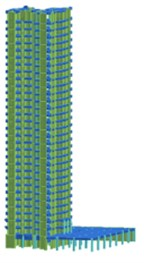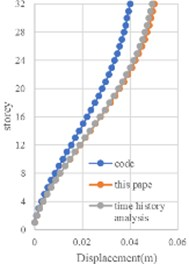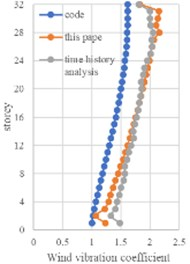# Mode-superposition response spectrum method for wind-induced vibration analysis of structures

## Yinfeng Dong1, Xu Huang2, Wengju Liang3, Dong Li4

1, 2, 3, 4Key Laboratory of Mountain Town Construction and New Technology of Ministry of Education (Chongqing University), Chongqing, 400045, China

1, 2, 3, 4School of Civil Engineering, Chongqing University, Chongqing, 400045, China

2Corresponding author

Vibroengineering PROCEDIA, Vol. 33, 2020, p. 113-117. https://doi.org/10.21595/vp.2020.21690
Received 13 September 2020; received in revised form 1 October 2020; accepted 8 October 2020; published 19 October 2020

47th International Conference on Vibroengineering in Timisoara, Romania, October 19-20, 2020

Copyright © 2020 Yinfeng Dong, et al. This is an open access article distributed under the Creative Commons Attribution License, which permits unrestricted use, distribution, and reproduction in any medium, provided the original work is properly cited.
Views 111
Abstract.

The calculation process of time history analysis method is complicated and time-consuming which is not convenient for wide application and promotion in engineering design. Based on the idea of mode decomposition in structural seismic response analysis, the fluctuating wind response spectrum and the mode decomposition response spectrum method for wind-induced vibration analysis are derived in this paper. Taking a high-rise building structure as an example, this paper uses the method proposed in this paper, the time history analysis method and the method of my country's load code to compare the wind-induced vibration response analysis. The results show that the method proposed in this paper is simple, effective and convenient for engineering application.

Highlights
• Based on the idea of mode decomposition in structural seismic response analysis, the fluctuating wind response spectrum and the mode decomposition response spectrum method for wind-induced vibration analysis are derived in this paper.
• This paper derives the relationship between the power spectral density function of the random process and the maximum absolute value of the process time domain.
• This paper uses the frequency response function of the single degree of freedom system to obtain the power spectrum of the single degree of freedom system response from the pulsating wind power spectrum.
• Compared with the time history analysis method and the Chinese load code method, the method in this paper is simpler, more effective, and more convenient for engineering application.

Keywords: wind, vibration, response spectrum, mode-superposition response spectrum method.

#### 1. Introduction

The time history analysis method to determine the wind vibration response of the structure requires multiple input wind load time history at the same time, and in order to ensure that the analysis result has a certain guarantee rate, a sufficient number of wind load input samples are required .Therefore, the calculation process of this method is complicated, the calculation amount is large, and it is not convenient for application and promotion in engineering design. For this reason, based on the idea of modal decomposition in structural seismic response analysis , this paper proposes and derives the modal decomposition response spectrum method for structural wind-induced response analysis, and uses a calculation example to verify the effectiveness of the method.

#### 2. The relationship between the power spectrum of a random process and its extreme value in the time domain

Let $s\left(t\right)$ denote the zero-mean stationary Gaussian process, ${G}_{s}\left(\omega \right)$ denote the unilateral power spectrum probability density function of the process, and within the period τ, the maximum absolute value ${S}_{t}$ of the random process $s\left(t\right)$ is defined as:${S}_{t}=\underset{t}{max}\left|s\left(t\right)\right|$, $0\le t\le \tau$.

According to the derivation of Vanmarcke [2-4], the probability distribution function of ${S}_{t}$ is:

(1)
${F}_{{s}_{\tau }}\left(s\right)=\left(1-{e}^{-\frac{{a}^{2}}{2}}\right)\mathrm{e}\mathrm{x}\mathrm{p}\left\{-\nu \tau {e}^{-\frac{{a}^{2}}{2}}\frac{1-{e}^{-\sqrt{\frac{\pi }{2}}}{q}_{e}a}{1-{e}^{-\frac{{a}^{2}}{2}}}\right\},$

where,$a=s/\sqrt{{\lambda }_{0}}\text{;}$$\nu$ is the average zero crossing rate,$q=\sqrt{1-{{\lambda }_{1}}^{2}/{\lambda }_{0}{\lambda }_{2}}$; $b$ is the empirical constant, which is generally set as 0.2; ${\lambda }_{m}$ is origin moment of power spectrum, ${\lambda }_{m}=\underset{0}{\overset{\infty }{\int }}{\omega }^{m}{G}_{s}\left(\omega \right)d\omega$ (0, 1, 2...), $\omega$ is the circular frequency.

Then the random variable ${S}_{t}$ is the expected maximum absolute value of the random process as:

(2)
${\mu }_{{s}_{\tau }}={\int }_{0}^{\infty }s{f}_{{s}_{\tau }}\left(s\right)ds,$

where, ${f}_{{s}_{\tau }}\left(s\right)$ is the probability density function of${S}_{t}$, $\frac{d{F}_{{s}_{\tau }}\left(s\right)}{ds}={f}_{{s}_{\tau }}\left(s\right)$. Correspondingly, the standard deviation of ${S}_{t}$ is:

(3)
${\sigma }_{{s}_{\tau }}=\sqrt{{\int }_{0}^{\infty }\left(s-{\mu }_{{s}_{\tau }}{\right)}^{2}{f}_{{s}_{\tau }}\left(s\right)ds}.$

#### 3. Response spectrum of a single degree of freedom system

For instantaneous wind speed $U\left(t\right)$, it can be decomposed into average wind speed ${U}_{0}$ and fluctuating wind speed $V\left(t\right)$. According to the tentative assumption, the pulsating wind speed is generally less than the average wind speed, so the smaller square term can be ignored, then:

(4)
${U}^{2}\left(t\right)={{U}_{0}}^{2}+2{U}_{0}V\left(t\right).$

Correspondingly, wind pressure and wind force are expressed as $W\left(t\right)={W}_{0}\left(1+2V\left(t\right)/{U}_{0}\right)$, $P\left(t\right)={P}_{0}\left(1+2V\left(t\right)/{U}_{0}\right)$, where ${W}_{0}={U}_{0}^{2}/1600$, ${P}_{0}={W}_{0}A$, $A$ is the wind-receiving area. The above formula shows that the wind force generated by the pulsating wind is $2V\left(t\right)/{U}_{0}$ times the wind force generated by the average wind.

If it is assumed that the ratio of the load generated by the average wind to the mass m of the single degree of freedom system is 1, and only the vibration caused by the pulsating wind is considered, the motion equation of a single degree of freedom system under wind load $P\left(t\right)$ is:

(5)
$\stackrel{¨}{x}+2\xi {\omega }_{0}\stackrel{˙}{x}+{{\omega }_{0}}^{2}x=\frac{2V\left(t\right)}{{U}_{0}}.$

Carry out Fourier transform on both sides of Eq. (5), set the Fourier transform of $x\left(t\right)$ and $V\left(t\right)$ to $F\left(\omega \right)$ and ${F}_{v}\left(\omega \right)$, and use $\mathcal{F}\left(.\right)$ to represent the Fourier transform, then $\mathcal{F}\left(\stackrel{˙}{x}\right)=i\omega \cdot F\left(\omega \right)$, $\mathcal{F}\left(\stackrel{¨}{x}\right)=\left(i\omega {\right)}^{2}\cdot F\left(\omega \right)$, $\mathrm{\Phi }\left(v\right)={F}_{v}\left(\omega \right)$. Putting the above equations into Eq. (5) to get:

(6)
$F\left(\omega \right)\left[-{\omega }^{2}+2\xi {\omega }_{0}\left(i\omega \right)+{{\omega }^{2}}_{0}\right]=\frac{2}{{U}_{0}}{F}_{v}\left(\omega \right).$

Let ${S}_{x}\left(\omega \right)$ be the power spectrum of the displacement response of the single degree of freedom system, and ${S}_{v}\left(\omega \right)$ be the power spectrum of the pulsating wind. According to the above Fourier spectrum, the relationship of power spectrum can be obtained [5, 6]:

${S}_{x}\left(\omega \right)\cdot {‖-{\omega }^{2}+2\xi {\omega }_{0}\left(i\omega \right)+{{\omega }^{2}}_{0}‖}^{2}=\frac{4}{{{U}^{2}}_{0}}{S}_{v}\left(\omega \right).$

Let${‖H\left(\omega \right)‖}^{2}=\frac{1}{\left({\omega }_{0}-\omega {\right)}^{2}+4{\xi }^{2}{{\omega }_{0}}^{2}{\omega }^{2}}$, $H\left(\omega \right)$ is the transfer function of the single-degree-of-freedom system, the relationship of the power spectrum is:

(7)
${S}_{x}\left(\omega \right)={‖H\left(\omega \right)‖}^{2}\cdot \frac{4}{{{U}^{2}}_{0}}\cdot {S}_{v}\left(\omega \right),$

the power spectrum of fluctuating wind adopts the Davenport spectrum ${S}_{v}\left(\omega \right)=4{\stackrel{-}{\omega }}^{2}{{v}_{*}}^{2}/\omega \left(1+\omega {\right)}^{4/3}$.

From Eq. (7), the power spectrum of the displacement response $x\left(t\right)$ of a single degree of freedom system can be obtained, and then the maximum absolute value of displacement response $x\left(t\right)$, i.e., ${S}_{d}$ can be obtained by Eqs. (2) and (3). By changing the natural frequency of the single degree of freedom system ${\omega }_{0}$, the corresponding curve of ${S}_{d}$ Vs. ${\omega }_{0}$ can be obtained, which is the displacement response spectrum corresponding to the fluctuating wind process. and other response spectra can be obtained by the Fourier transform conversion mentioned above. Of course, the response spectrum here is a standardized value assuming that the ratio of the load generated by the average wind to the mass of the single-degree-of-freedom system is 1, which must be multiplied by the value ${P}_{0}/m$ in practical applications.

#### 4. Mode decomposition response spectrum method for wind-induced response analysis of multi-degree-of-freedom (MDOF) systems

The motion equation of a MDOF system under wind load is:

(8)
$\left[\mathbf{M}\right]\left\{\stackrel{¨}{x}\left(t\right)\right\}+\left[\mathbf{C}\right]\left\{\stackrel{˙}{x}\left(t\right)\right\}+\left[\mathbf{K}\right]\left\{x\left(t\right)\right\}=\left\{P\left(t\right)\right\}.$

If only the influence of pulsating wind is considered:

(9)
$\left\{P\left(t\right)\right\}=\left\{{P}_{i}\left(t\right)\right\}=\left\{{W}_{i}{A}_{i}\frac{2{V}_{i}\left(t\right)}{{U}_{i}}\right\}=\left\{{W}_{0}{\mu }_{{s}_{i}}\left(\frac{{z}_{i}}{10}{\right)}^{\alpha }{\mu }_{R}\cdot {A}_{i}\cdot \frac{2{V}_{i}\left(t\right)}{{U}_{0}}\right\},$
(10)
${C}_{{p}_{i}}={W}_{0}{\mu }_{{s}_{i}}{\left(\frac{{z}_{i}}{10}\right)}^{\alpha }{\mu }_{R}\cdot {A}_{i},$
(11)
$\left\{{P}_{i}\left(t\right)\right\}=\left\{{W}_{i}{A}_{i}\frac{2{V}_{i}\left(t\right)}{{U}_{i}}\right\}=\left\{{C}_{{p}_{i}}\cdot \frac{2{V}_{i}\left(t\right)}{{U}_{0}}\right\},$

where, ${W}_{0}$ is the basic wind pressure; ${U}_{i}$ is the average wind speed at the mass point; ${\mu }_{{s}_{i}}$ is the body shape coefficient at the $i$-th mass point; $\alpha$ is the ground roughness index; ${\mu }_{R}$ is the ground roughness correction coefficient, for A~D ground roughness category taking 1.284, 1.000, 0.544 and 0.262 respectively; ${A}_{i}$ is the wind area.

For a MDOF system, the orthogonality of the stiffness matrix, damping matrix, and mass matrix of the mode vector can be used to decouple the motion equations of the MDOF system and convert it into the motion of a single-degree-of-freedom system to obtain the corresponding. After that, the mode superposition is performed to obtain the response of the MDOF system. The displacement of a MDOF system can be expressed by the corresponding mode shape, namely $\left\{x\left(t\right)\right\}=\left[\varphi \right]\left\{q\left(t\right)\right\}$.

Substituting equation $\left\{x\left(t\right)\right\}=\left[\varphi \right]\left\{q\left(t\right)\right\}$ into Eq. (8), and multiplying it by the transposition $\left\{{\varphi }_{j}{\right\}}^{T}$ of the mode vector, a series of independent equations can be obtained from the orthogonality of the mode shape. Each equation can be written as:

(12)
${\stackrel{¨}{q}}_{j}\left(t\right)+2{\xi }_{j}{\omega }_{j}{\stackrel{˙}{q}}_{j}\left(t\right)+{{\omega }^{2}}_{j}{q}_{j}\left(t\right)={F}_{j}\left(t\right),$

where, ${F}_{j}\left(t\right)={{p}_{j}}^{*}\left(t\right)/{{m}^{*}}_{j}=\sum _{i=1}^{n}{\varphi }_{ij}{P}_{j}\left(t\right)/{{m}^{*}}_{j}$. Similar to the case of a single-degree-of-freedom system, the Fourier transformed on both sides of Eq. (12) and converted into the corresponding power spectrum relationship can be obtained:

(13)

Eq. (12) is the equation of motion for a single degree of freedom system corresponding to the generalized coordinates. Therefore, only the power spectrum required can be used to find the time domain maximum value of the random process using the previous Eqs. (2) and (3). From Eqs. (10-13), we can find:

(14)
${S}_{{q}_{j}{q}_{j}}\left(\omega \right)=\frac{{{\left\{{C}_{s}\right\}}^{T}}_{j}\left[\mathbf{R}\right]\left\{{C}_{\mathbit{s}}\right\}}{{m}_{j}^{*}\cdot {m}_{j}^{*}}\cdot {‖H\left(\omega \right)‖}^{2}\cdot \frac{4}{{{U}^{2}}_{0}}\cdot {S}_{v}\left(\omega \right),$
(15)
(16)
${S}_{{q}_{j}{q}_{j}}\left(\omega \right)={C}_{{F}_{j}}\cdot {‖H\left(\omega \right)‖}^{2}\cdot \frac{4}{{{U}^{2}}_{0}}\cdot {S}_{v}\left(\omega \right),$

where, $\left[\mathbf{R}\right]$ is the correlation coefficient matrix corresponding to the multi-particle pulsating wind spectrum, and ${R}_{ij}\left(\omega \right)=\mathrm{e}\mathrm{x}\mathrm{p}\left[-C\left(\omega \left|{z}_{i}-{z}_{j}\right|/2\pi \stackrel{-}{U}\right)\right]$, among them, $\left|{z}_{i}-{z}_{j}\right|$ is the distance between the two points $i$ and $j$, $\omega$ is the circular frequency, $\stackrel{-}{U}$ is the average wind speed between the two points $i$ and $j$, $C$ is the attenuation coefficient, usually taken 10-20, this article takes 10.

Accordingly:

(17)
${\left|{q}_{j}\left(t\right)\right|}_{max}=\sqrt{{C}_{{F}_{j}}}\cdot {S}_{d}\left({\omega }_{j}\right)=\sqrt{{C}_{{F}_{j}}}\cdot {S}_{d}\left({T}_{j}\right).$

For the $j$-th mode, the response of the MDOF system is:

(18)
${\left\{x\right\}}_{j}=\left\{{\varphi }_{ji}\right\}\cdot {\left|{q}_{j}\left(t\right)\right|}_{max}=\left\{{\varphi }_{ji}\right\}\cdot \sqrt{{C}_{{F}_{j}}}\cdot {S}_{d}\left({T}_{j}\right).$

After finding the responses of all modes, use the “Sum of Squares Method” (SRSS) to find the total response, i.e., $\left\{x\right\}=\sum _{j=1}^{n}\sqrt{\left\{x{{\right\}}^{2}}_{j}}$.

#### 5. Example analysis

Taking a 32-story high-rise building as an example (Fig. 1(a)), under the same model and conditions the response of the structure under the action of average divided wind and fluctuating wind was calculated using the method of this paper, the time history analysis method and the method specified in the load code. According to the design conditions, the basic wind pressure is 0.3 kN/m2 in the calculation, the ground roughness category is Class B, and the result of the time history analysis is the average of the calculation results of 10 synthetic pulsating wind samples.

Fig. 1(b) and (c) show the floor displacement and wind vibration coefficient of each floor of the structure calculated by three methods. It can be seen that the calculation results of the time history analysis method and the method in this paper are very close, and the relative error can be ignored; the calculation result of the standard method is too small. The maximum difference in floor displacement is about 25 %, and the maximum difference in wind vibration coefficient is about 0.6. It shows that for relatively high-flexible structures, in accordance with the requirements of the load code, the wind vibration coefficient is used to consider the influence of fluctuating wind, and the calculation results of the structure’s wind vibration response may be underestimated.

Fig. 1. Comparison of story displacement and dynamic response factor obtained by using three methods for MDOF structure: a) tall building used as an example; b) displacement response; c) wind vibration coefficienta)b)c)

#### 6. Conclusions

1) Given the power spectrum of pulsating wind, the frequency response function of the single degree of freedom system is used to obtain the power spectrum of the single degree of freedom system response. From this, the time domain maximum value of the single degree of freedom system response is derived. And then the relationship curve between the natural frequency and the maximum response of a single degree of freedom system can be obtained, which is the pulsating wind response spectrum.

2) Based on the idea of mode decomposition, the mode decomposition response spectrum method for structural wind-induced response analysis is deduced, and a high-rise building example is used to verify its effectiveness. The results show that the method in this paper is simple, effective and convenient for engineering applications.

1. Chopra A. K. Dynamics of Structures: Theory and Applications to Earthquake Engineering. Englewood Cliffs, Prentice Hall, 1995. [Search CrossRef]
2. Vanmarcke E. H. On the distribution of the first-passage time for normal stationary random processes. Journal of Applied Mechanics, Vol. 42, Issue 1, 1975, p. 215-220. [Publisher]
3. Vanmarcke E. H. Random Fields: Analysis and Synthesis. Cambridge, MA: MIT Press, 1983. [Search CrossRef]
4. Kiureghian A. D. On Response of Structures to Stationary Excitation. Report No. EERC 79/32, Berkeley, California, 1979. [Search CrossRef]
5. Li Jie, Zhang Linlin A study on the relationship between turbulence power spectrum and stochastic Fourier amplitude spectrum. Journal of Disaster Prevention and Mitigation Engineering, Vol. 4, 2004, p. 363-369, (in Chinese). [Search CrossRef]
6. Montejo L. A., Vidot Vega A.-L. An empirical relationship between Fourier and response spectra using spectrum-compatible times series. Earthquake Spectra, Vol. 33, Issue 1, 2017, p. 179-199. [Publisher]

#### Cited By

 Mochammad Faqih, Nu Rhahida Arini, Hendrik Elvian Gayuh Prasetya, Bahashwan Abdulrahman Abdullah Omar, Temitope Ibrahim Amosa 2022